# Chapter 12 Single Boxplot

For boxplots with no outlier, we will use the dataset, ldeaths, which is a dataset built into R. Note that ldeaths is a vector. To see a description of this dataset, type ?ldeaths. A description will appear on the 4th panel under the Help tab.

To view the whole dataset, use the command View(ldeaths). A column of observations will appear on the Source panel, under the tab called ldeaths. You should see 1 column with 72 entries.

For boxplots with at least one outlier, we will be using the dataset called UScereal that is found in the package, MASS. Most likely, MASS is already installed. If not, install it first, then load the package MASS.

#Load package MASS
library(MASS)

To see a description of the dataset, type ?UScereal. A description of the dataset will appear on the 4th panel under the Help tab. Note that UScereal is a data frame.

To view the whole dataset, use the command View(UScereal). A column of observations will appear on the Source panel, under the tab called UScereal.

## 12.1 Basic R Boxplot

To draw a boxplot in basic R, we use the function boxplot(quantitative_variable). The default boxplot is a vertical boxplot.

### Boxplot with No Outlier

Let us draw the boxplot for the dataset, ldeaths.

boxplot(ldeaths,
main = "Monthly Deaths from Lung Diseases in the UK",
ylab = "Number of Deaths")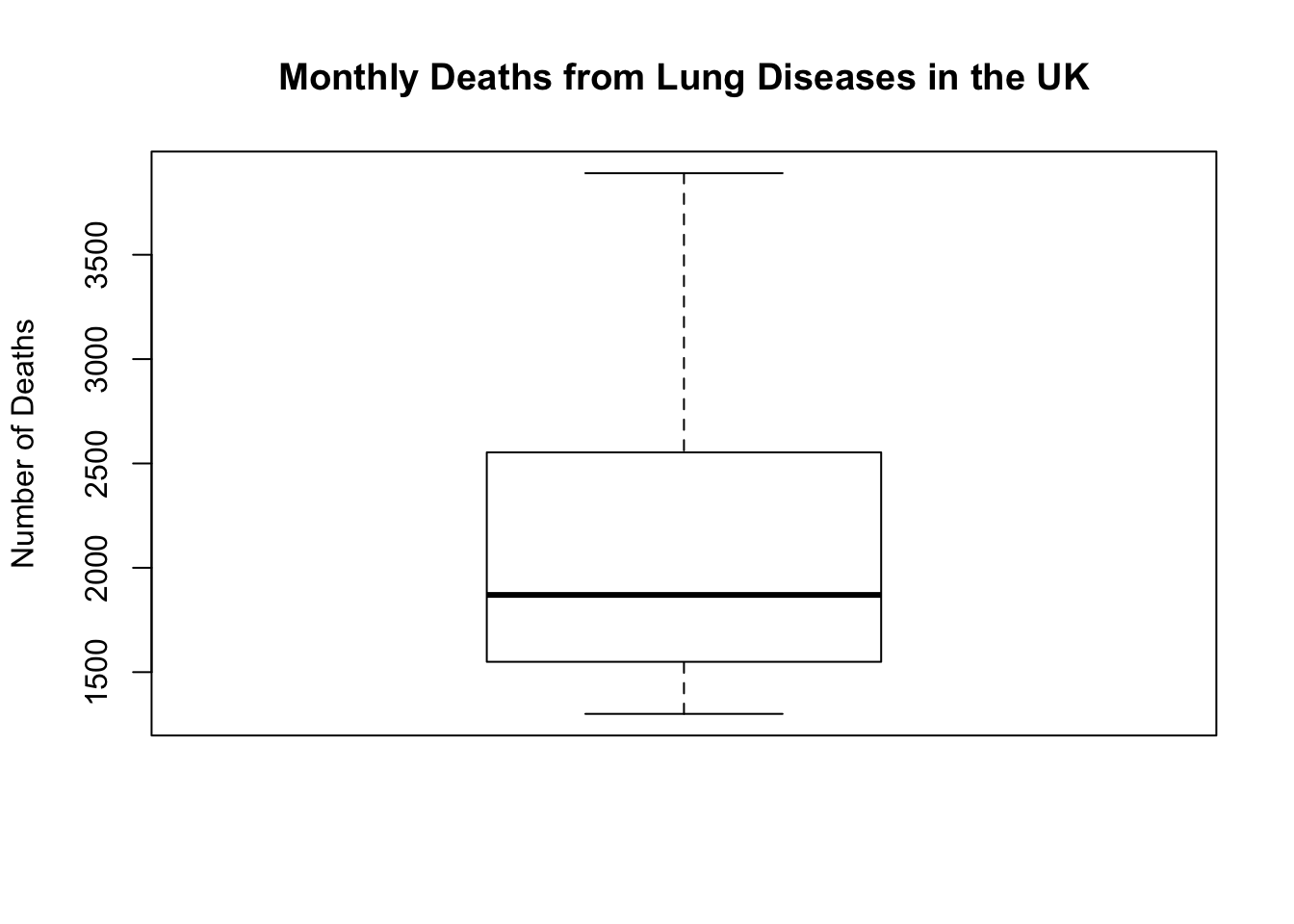To draw a horizontal boxplot, add the argument “horizontal = TRUE”.

boxplot(ldeaths,
main = "Monthly Deaths from Lung Diseases in the UK",
xlab = "Number of Deaths",
horizontal = TRUE)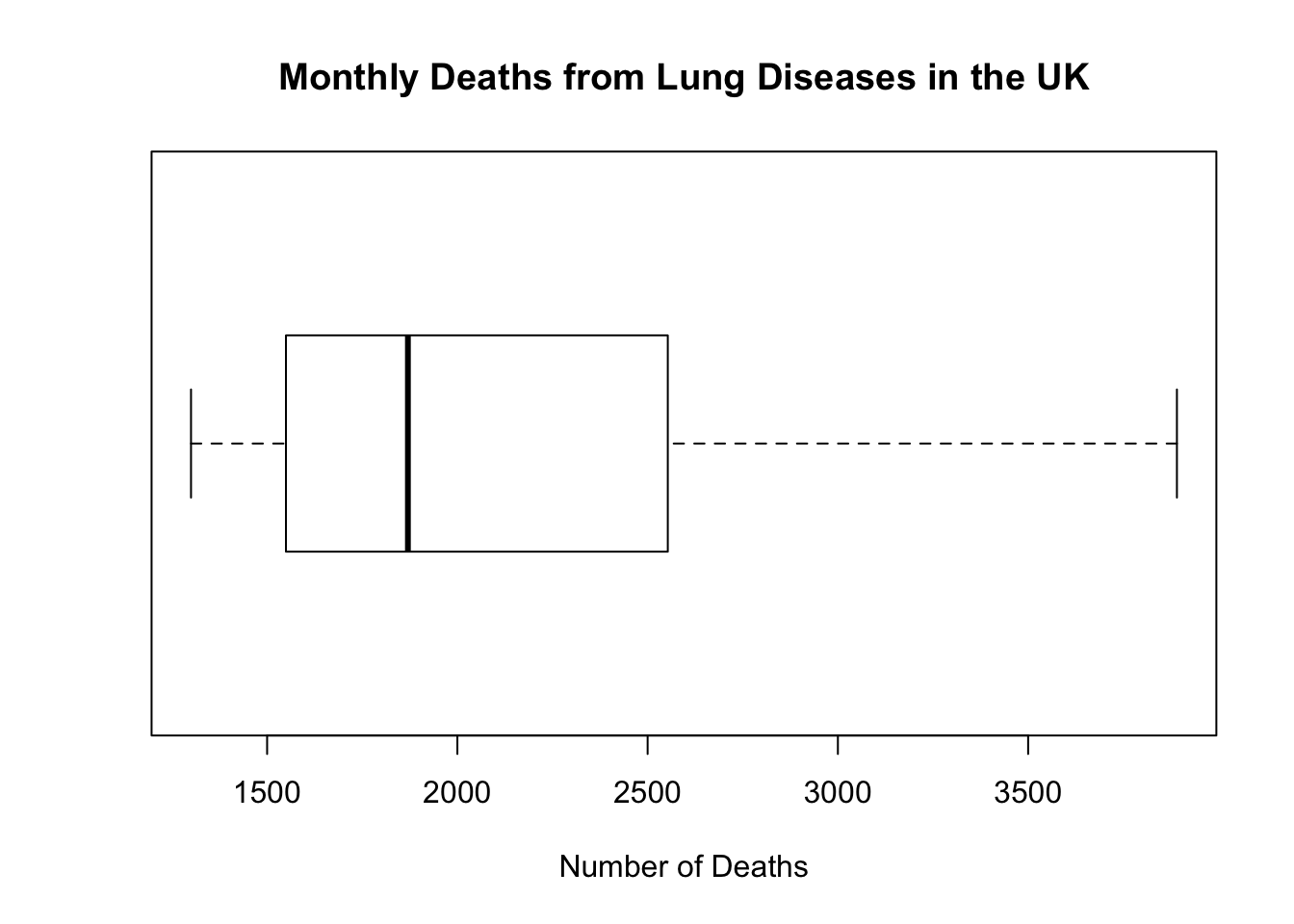Let us draw the histogram and stemplot and compare the results with the boxplot.

hist(ldeaths,
main = "Monthly Deaths from Lung Diseases in the UK",
xlab = "Number of Deaths")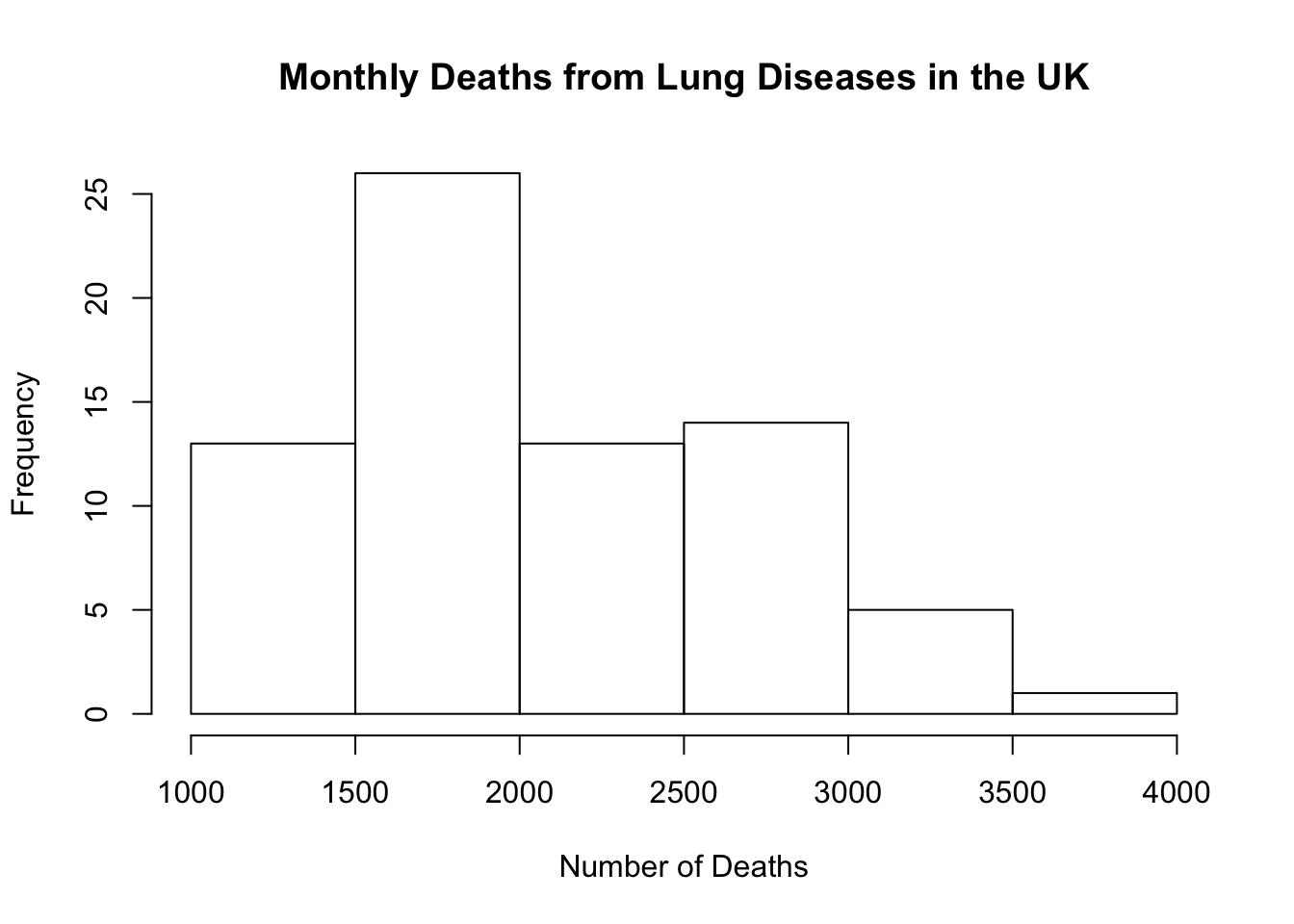stem(ldeaths)
##
##   The decimal point is 3 digit(s) to the right of the |
##
##   1 | 333444444
##   1 | 55555555566666666677777788999
##   2 | 000011123344
##   2 | 5556666778888999
##   3 | 01112
##   3 | 9

The three graphs are consistent with each other. All show a right-skewed distribution of deaths.

### Boxplot with Outlier

The dataset, UScereal, has several variables. We will focus on the variable, sodium, and draw its boxplot.

boxplot(UScereal\$sodium,
main = "Sodium Content in One Cup of US Cereal",
ylab = "Sodium Content (in milligrams)")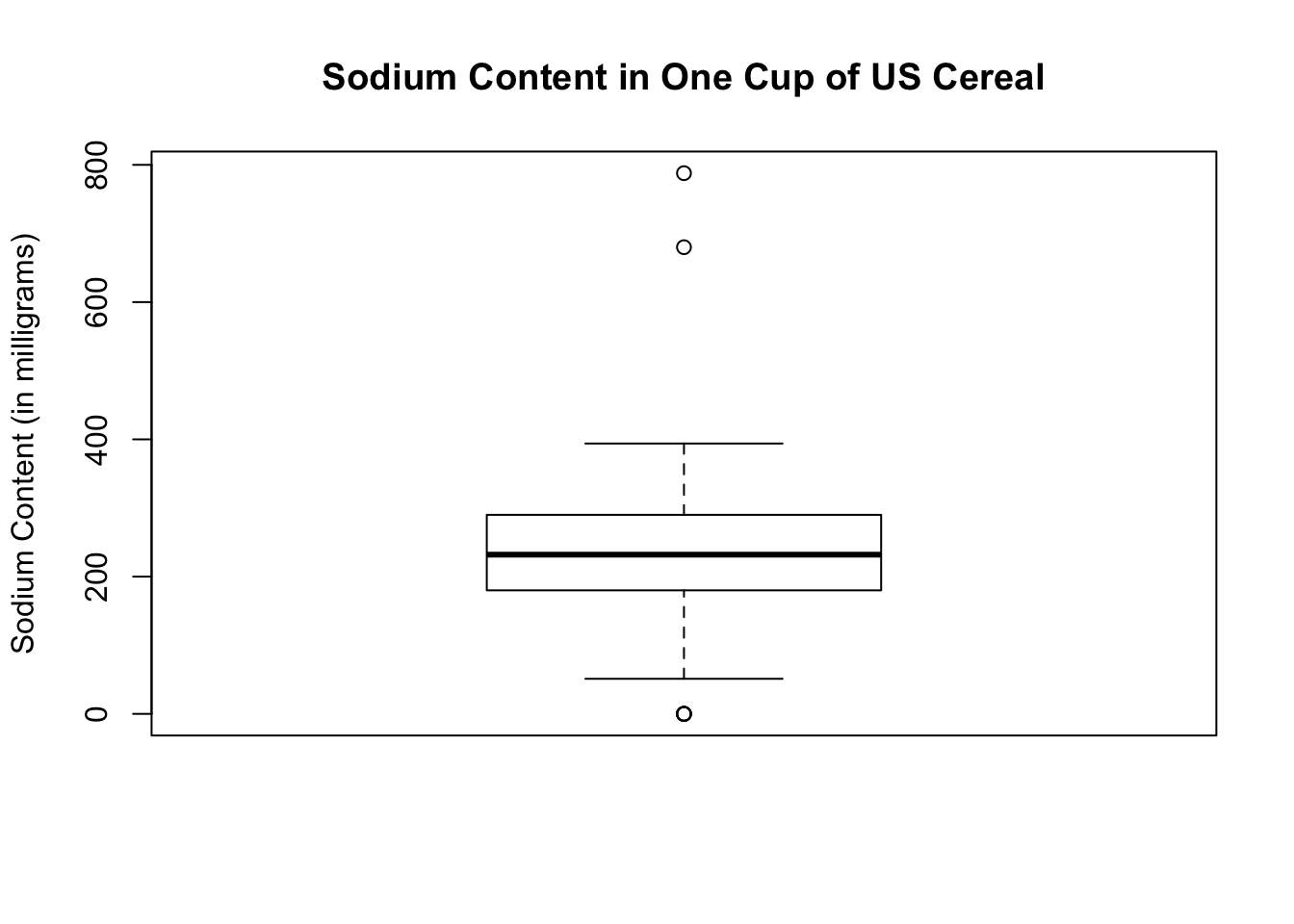The boxplot shows 3 outliers, one below the lower fence and two above the upper fence.

## 12.2 Ggplot2 Boxplot

# Load ggplot2
library(ggplot2)

Here are some of the basic commands used to draw a boxplot in ggplot2.

• ggplot(data = data_frame, aes (y = vector)) – initializes a ggplot object
• geom_boxplot( ) – geometric shape to make a boxplot
• scale_x_discrete( ) - leave the argument empty to remove extraneous numbers on the x-axis and to contract the boxplot otherwise the boxplot is very wide
• lab( ) - for labelling
• coord_flip( ) - draws a horizontal boxplot

### Boxplot with No Outlier

Remember that our dataset, ldeaths, is a vector. There is no need to put any arguments in the function ggplot( ).

ggplot() +
geom_boxplot(aes(y = ldeaths)) +
scale_x_discrete( ) +
labs(title = "Monthly Deaths from Lung Diseases in the UK",
y = "Number of Deaths")
## Don't know how to automatically pick scale for object of type ts. Defaulting to continuous.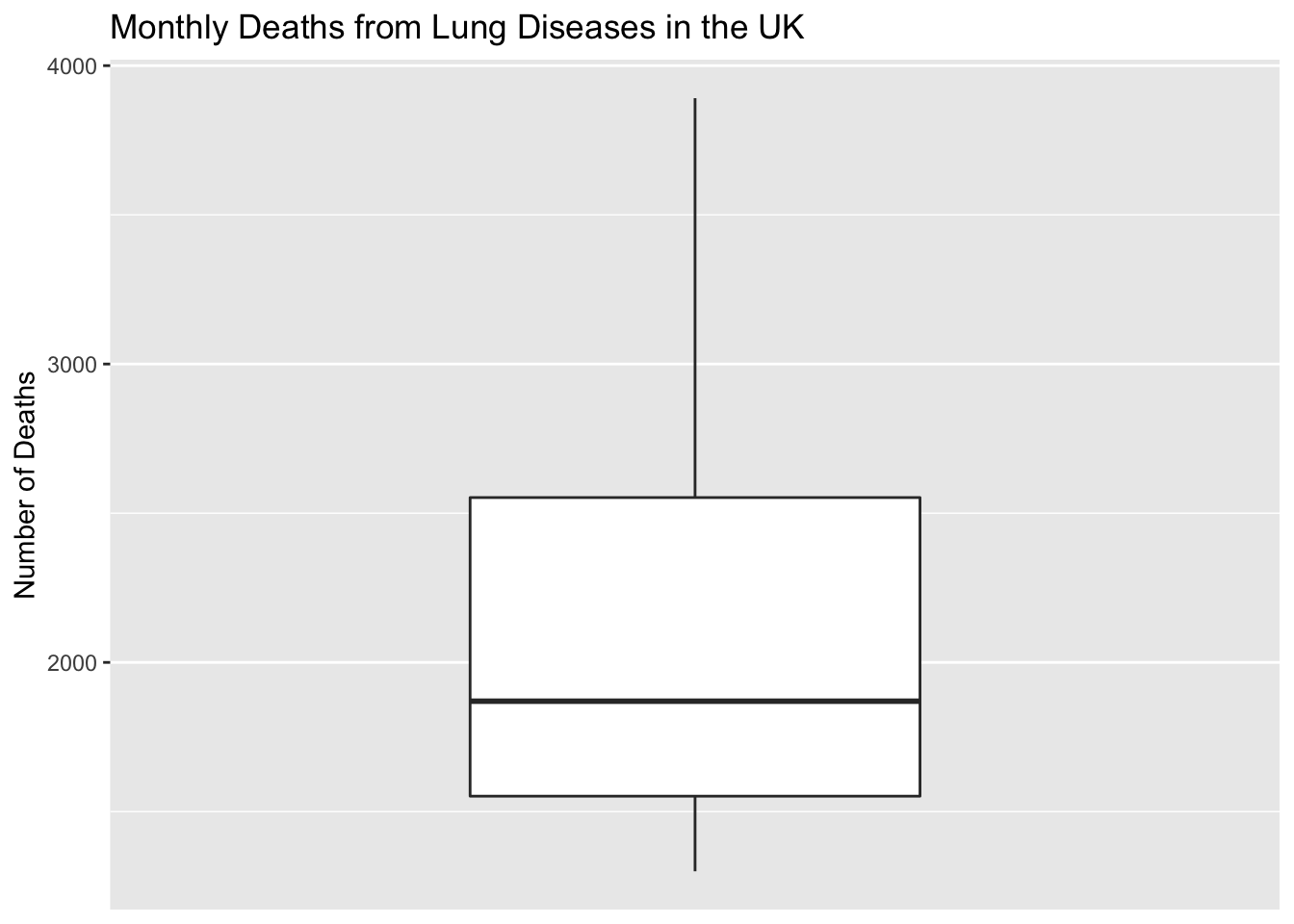Notice there is a message regarding the boxplot scale. Boxplots are usually drawn starting at y = 0. In this case, the boxplot does not start at y = 0. We see a jump in the scale. To fix the problem so the message does not appear, set the y-scale range using the argument ylim. For this particular dataset, we want the y-axis to go from 1000 to 4000.

ggplot() +
geom_boxplot(aes(y = ldeaths)) +
scale_x_discrete( ) +
ylim(c(1000, 4000)) +
labs(title = "Monthly Deaths from Lung Diseases in the UK",
y = "Number of Deaths")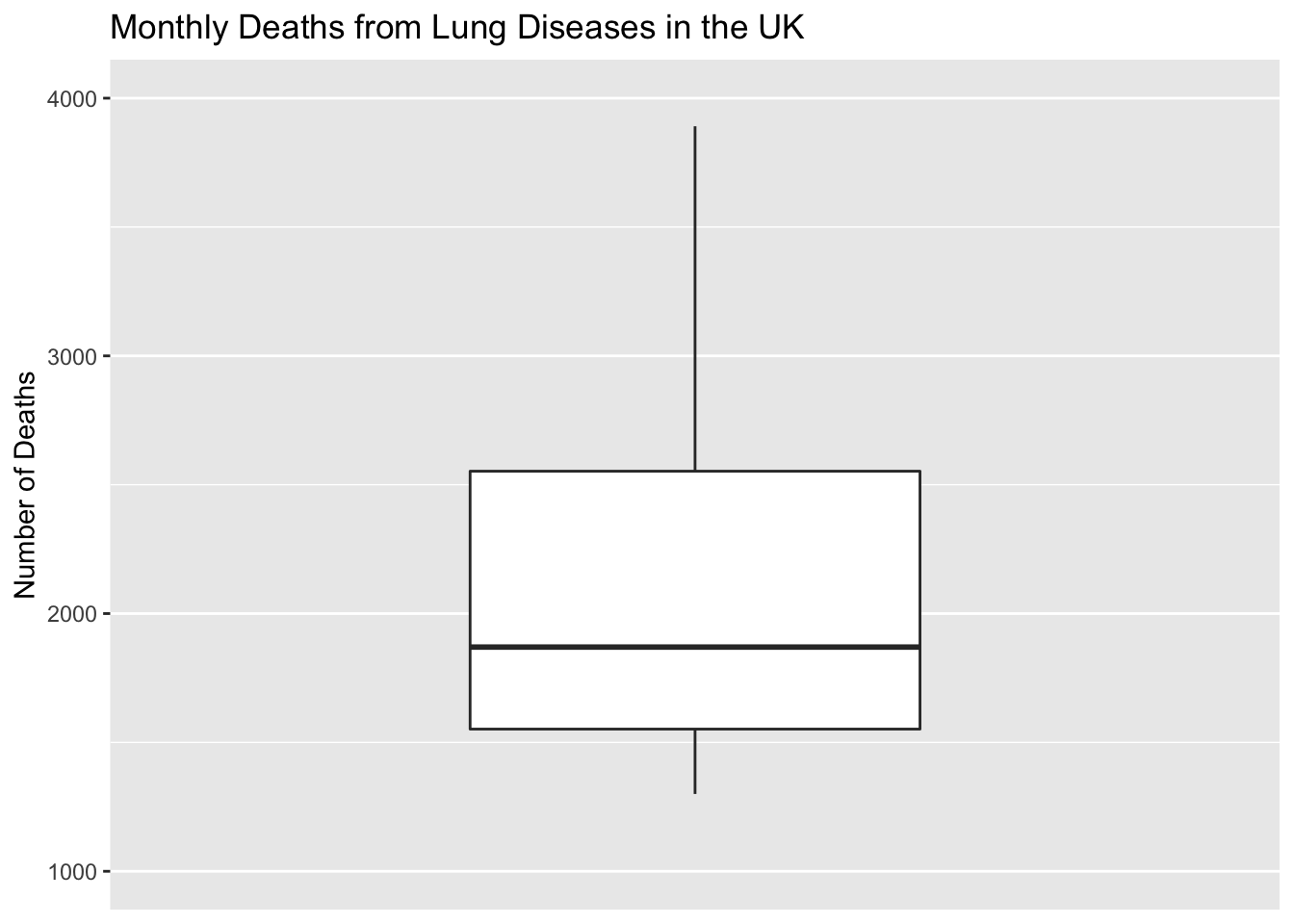Note that in ggplot2, the boxplot is drawn without whiskers by default. You can add whiskers but they do not look as nice as the whiskers in basic R. We will, therefore, not put any whiskers.

To draw a horizontal boxplot, add the command coord_flip( ).

ggplot() +
geom_boxplot(aes(y = ldeaths)) +
scale_x_discrete( ) +
ylim(c(1000, 4000)) +
labs(title = "Monthly Deaths from Lung Diseases in the UK",
y = "Number of Deaths") +
coord_flip()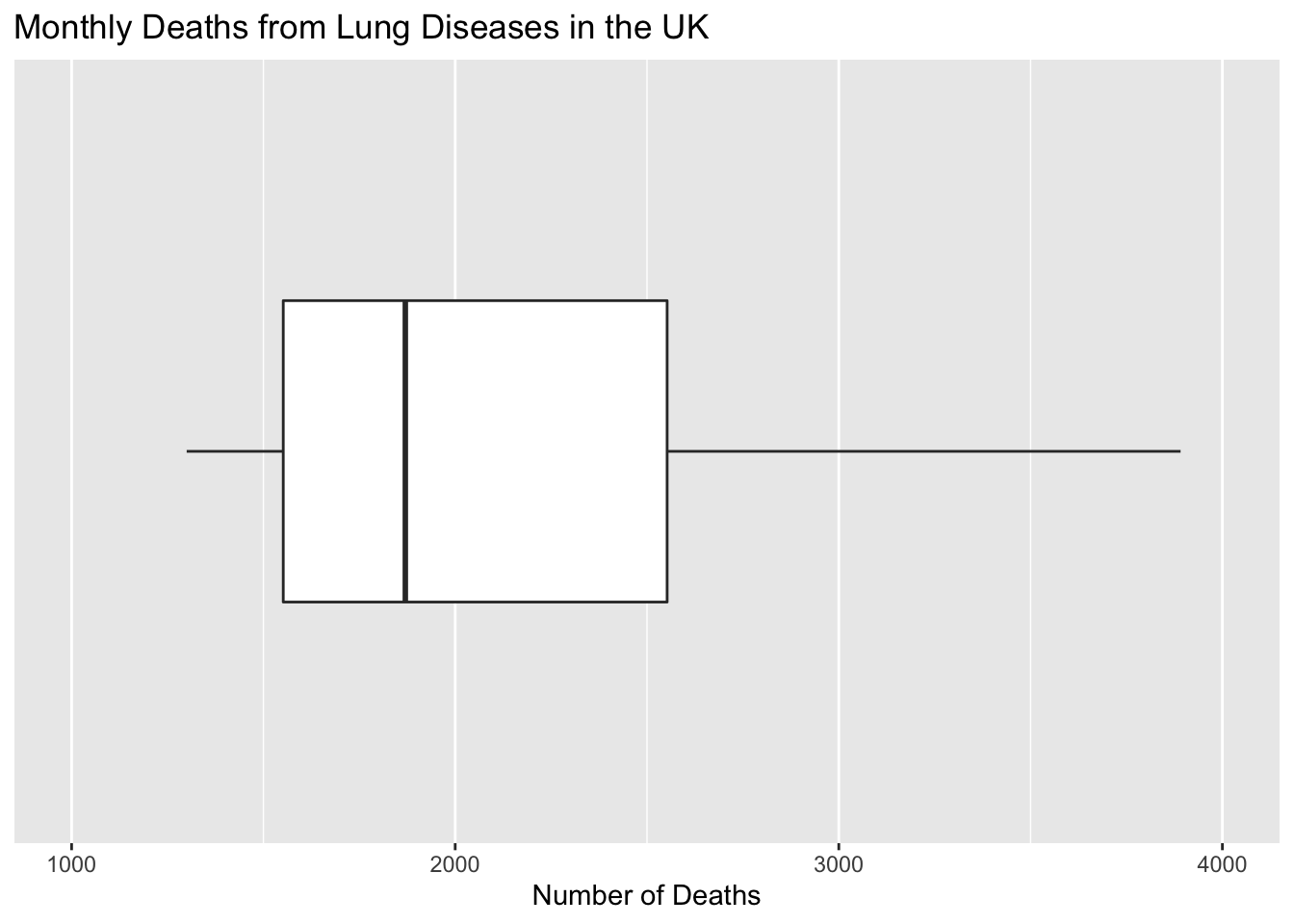### Boxplot with Outlier

Our dataset, UScereal, is a data frame and not a vector. Therefore, we need to specify the data frame and aesthetic mappings in the ggplot( ) function.

ggplot(data = UScereal, aes(y = sodium)) +
geom_boxplot() +
scale_x_discrete() +
labs(title = "Sodium Content in One Cup of US Cereal",
y = "Sodium Content (in milligrams)")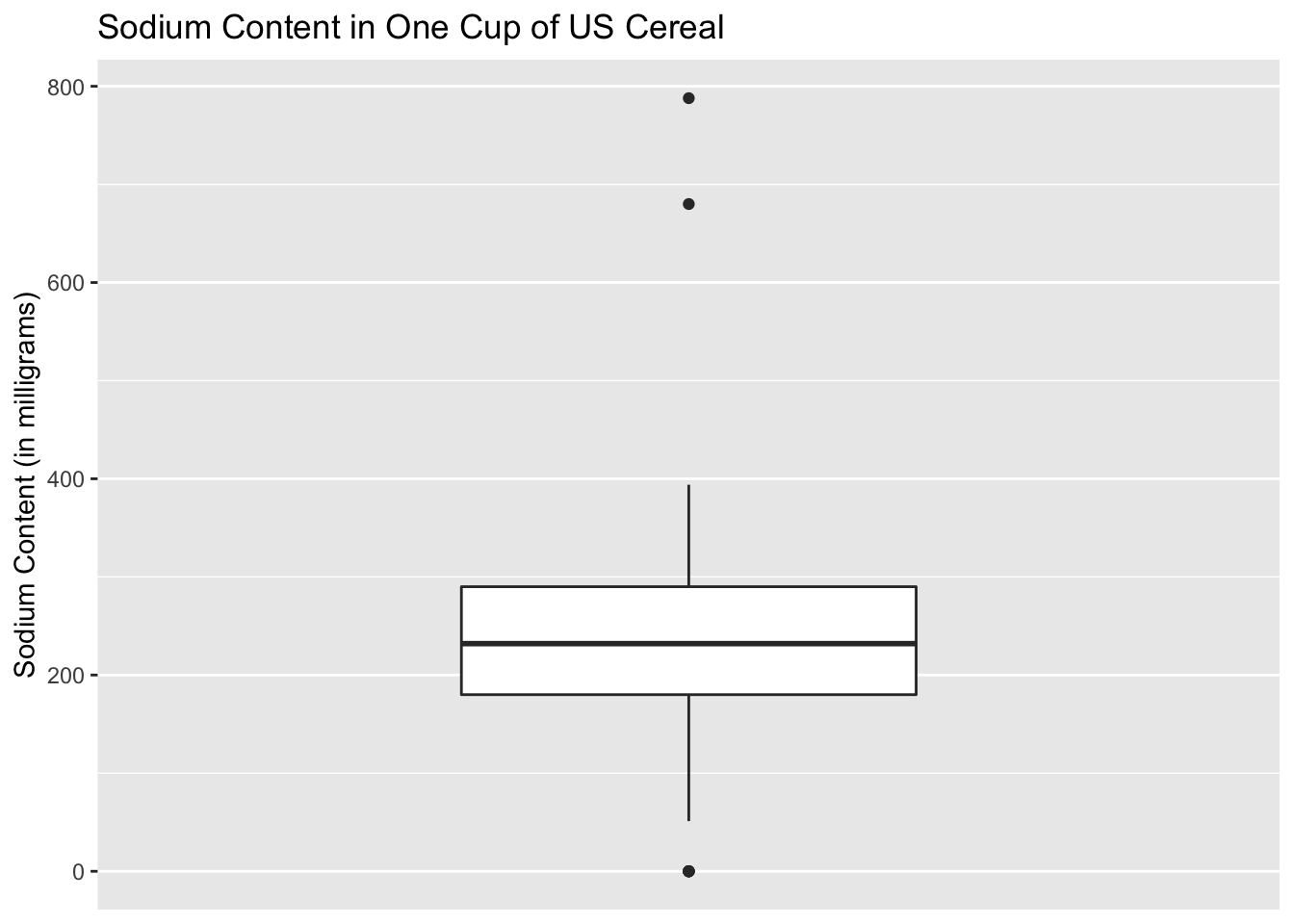The boxplot is consistent with that drawn in basic R. Both boxplots show three outliers.